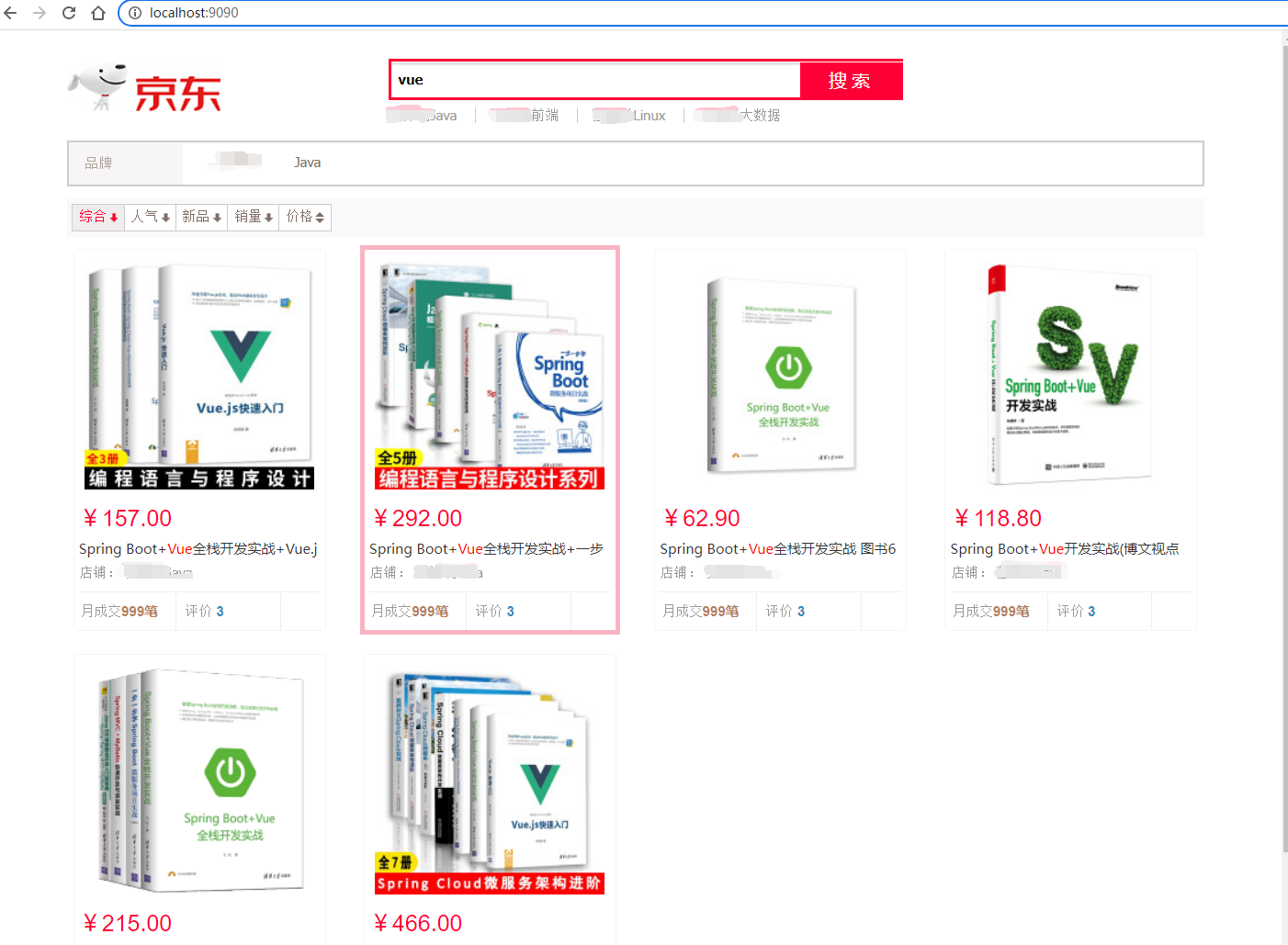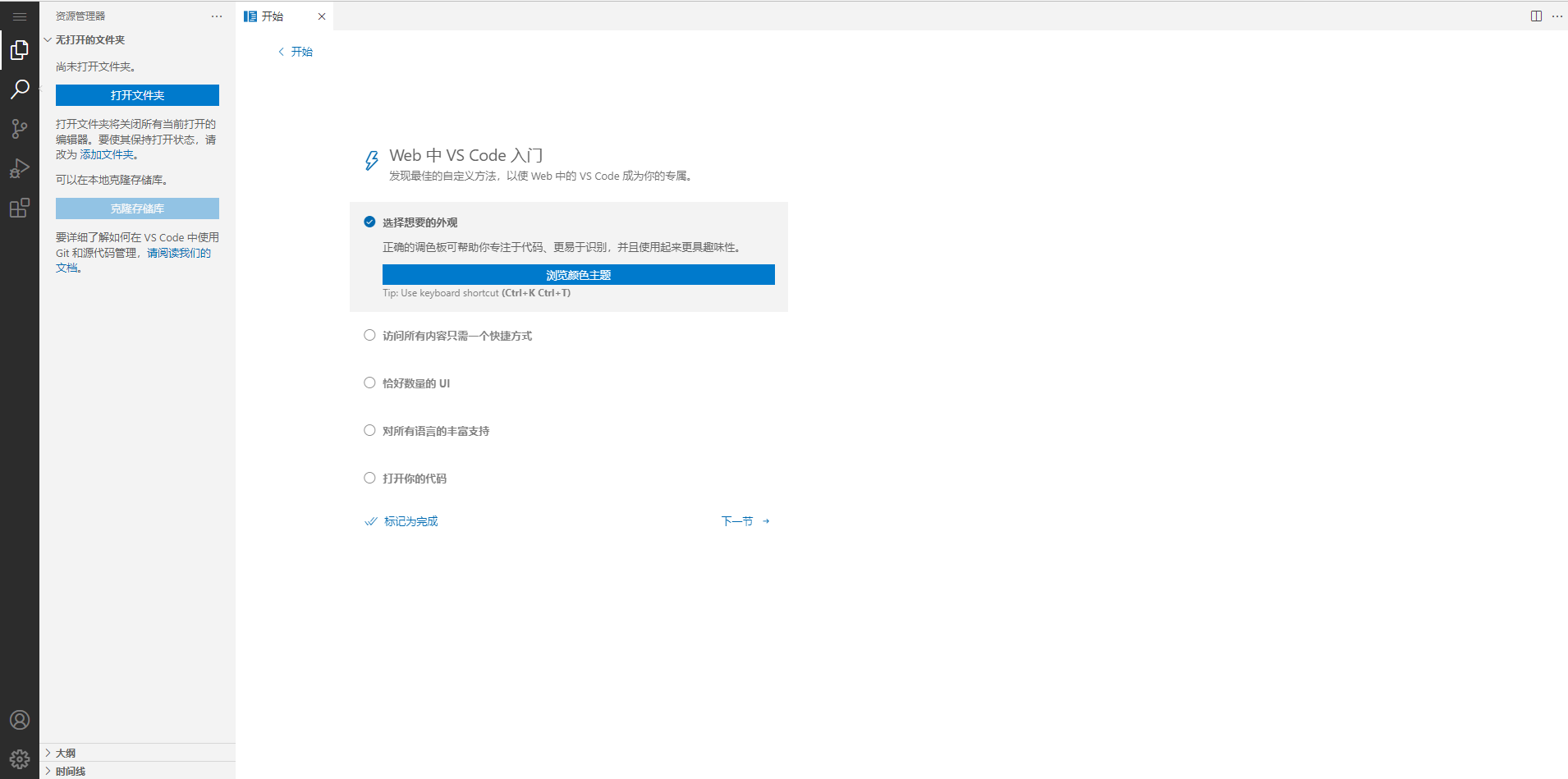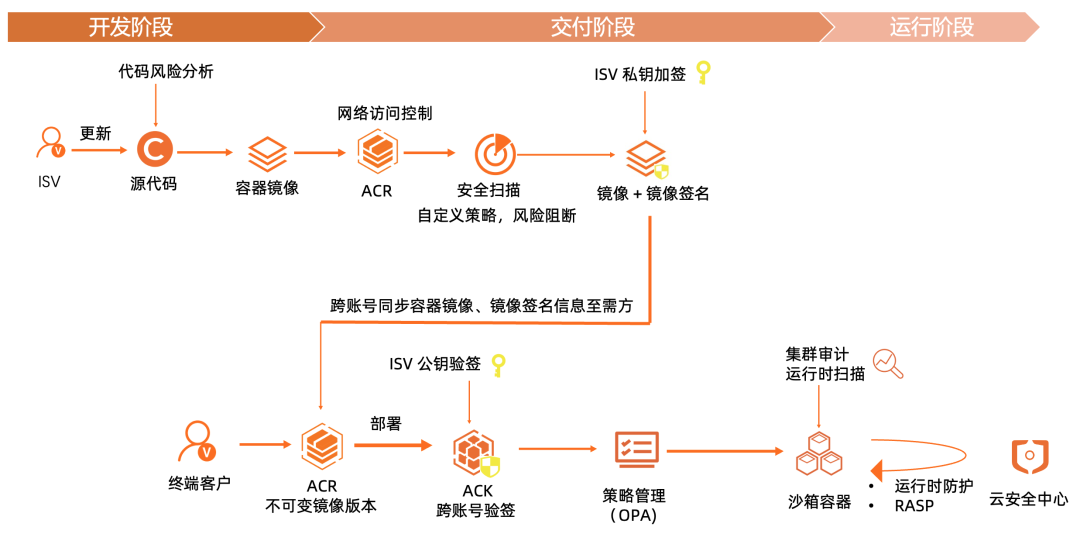# 了解JavaScript函数式编程（二）

+关注继续查看2.
3. foo == document.getElementById('foo');
4.
5. //=> false
6.
7. foo == document.getElementById('foo');
8.
9. //=> true

1. var Container = function(x) {
2.
3. this.__value = x;
4.
5.
6. Container.of = x => new Container(x);
7.
8. //试试看
9.
10. Container.of(1);
11.
12. //=> Container(1)
13.
14. Container.of('abcd');
15.
16. //=> Container('abcd'

1. Container.prototype.map = function(f){
2.
3. return Container.of(f(this.__value))
4.

1. Container.of(3)
2.
3. .map(x => x + 1) //=> Container(4)
4.
5. .map(x => 'Result is ' + x); //=> Container('Result is 4'

Functor(函子)是实现了 map 并遵守一些特定规则的容器类型。

1. var Maybe = function(x) {
2.
3. this.__value = x;
4.
5.
6. Maybe.of = function(x) {
7.
8. return new Maybe(x);
9.
10.
11. Maybe.prototype.map = function(f) {
12.
13. return this.isNothing() ? Maybe.of(null) : Maybe.of(f(this.__value));
14.
15.
16. Maybe.prototype.isNothing = function() {
17.
18. return (this.__value === null || this.__value === undefined);
19.
20.
21. //试试看
22.
23. import _ from 'lodash'
24.
26.
27. Maybe.of({name"Stark"})
28.
29. .map(_.prop("age"))
30.
32.
33. //=> Maybe(null
34.
35. Maybe.of({name"Stark", age: 21})
36.
37. .map(_.prop("age"))
38.
40.
41. //=> Maybe(31)

1. import _ from 'lodash'
2.
3. var compose = _.flowRight;
4.
6.
7. // 创造一个柯里化的 map
8.
9. var map = _.curry((f, functor) => functor.map(f));
10.
11. var doEverything = map(compose(add(10), _.property("age")));
12.
13. var functor = Maybe.of({name"Stark", age: 21});
14.
15. doEverything(functor);
16.
17. //=> Maybe(31)

1. try{
2.
3. doSomething();
4.
5. }catch(e){
6.
7. // 错误处理
8.

try/catch/throw 并不是“纯”的，因为它从外部接管了我们的函数，并且在这个函数出错时抛弃了它的返回值。这不是我们期望的函数式的行为。

1. doSomething()
2.
3. .then(async1)
4.
5. .then(async2)
6.
7. .catch(e => console.log(e));

1. // 这里是一样的=。=
2.
3. var Left = function(x) {
4.
5. this.__value = x;
6.
7.
8. var Right = function(x) {
9.
10. this.__value = x;
11.
12.
13. // 这里也是一样的=。=
14.
15. Left.of = function(x) {
16.
17. return new Left(x);
18.
19.
20. Right.of = function(x) {
21.
22. return new Right(x);
23.
24.
25. // 这里不同!!!
26.
27. Left.prototype.map = function(f) {
28.
29. return this;
30.
31.
32. Right.prototype.map = function(f) {
33.
34. return Right.of(f(this.__value));
35.

1. Right.of("Hello").map(str => str + " World!");
2.
3. // Right("Hello World!"
4.
5. Left.of("Hello").map(str => str + " World!");
6.
7. // Left("Hello"

Left 和 Right 唯一的区别就在于 map 方法的实现，Right.map 的行为和我们之前提到的 map 函数一样。但是 Left.map 就很不同了：它不会对容器做任何事情，只是很简单地把这个容器拿进来又扔出去。这个特性意味着，Left 可以用来传递一个错误消息。

1. var getAge = user => user.age ? Right.of(user.age) : Left.of("ERROR!");
2.
3. //试试
4.
5. getAge({name'stark', age: '21'}).map(age => 'Age is ' + age);
6.
7. //=> Right('Age is 21'
8.
9. getAge({name'stark'}).map(age => 'Age is ' + age);
10.
11. //=> Left('ERROR!'

Left 和 Right 是 Either 类的两个子类，事实上 Either 并不只是用来做错误处理的，它表示了逻辑或，范畴学里的coproduct。但这些超出了我们的讨论范围。

2.
3.   return window.localStorage;
4.

2.
3.   return function(){
4.
5.     return window.localStorage;
6.
7.   }

1. import _ from 'lodash'
2.
3. var compose = _.flowRight;
4.
5. var IO = function(f) {
6.
7. this.__value = f;
8.
9.
10. IO.of = x => new IO(_ => x);
11.
12. IO.prototype.map = function(f) {
13.
14. return new IO(compose(f, this.__value))
15.
16. };

IO 跟前面那几个 functor 不同的地方在于，它的 __value 是一个函数。它把不纯的操作(比如 IO、网络请求、DOM)包裹到一个函数内，从而延迟这个操作的执行。所以我们认为，IO 包含的是被包裹的操作的返回值。

1. var io_document = new IO(_ => window.document);
2.
3. io_document.map(function(doc){ return doc.title });
4.
5. //=> IO(document.title)

1. import _ from 'lodash'
2.
3. // 先来几个基础函数：
4.
5. // 字符串
6.
7. var split = _.curry((char, str) => str.split(char));
8.
9. // 数组
10.
11. var first = arr => arr;
12.
13. var last = arr => arr[arr.length - 1];
14.
15. var filter = _.curry((f, arr) => arr.filter(f));
16.
17. //注意这里的 x 既可以是数组，也可以是 functor
18.
19. var map = _.curry((f, x) => x.map(f));
20.
21. // 判断
22.
23. var eq = _.curry((x, y) => x == y);
24.
25. // 结合
26.
27. var compose = _.flowRight;
28.
29. var toPairs = compose(map(split('=')), split('&'));
30.
31. // toPairs('a=1&b=2'
32.
33. //=> [['a''1'], ['b''2']]
34.
35. var params = compose(toPairs, last, split('?'));
36.
37. // params('http://xxx.com?a=1&b=2'
38.
39. //=> [['a''1'], ['b''2']]
40.
41. // 这里会有些难懂=。= 慢慢看
42.
43. // 1.首先我们先对 url 调用 params 函数，得到类似[['a''1'], ['b''2']]
44.
45. // 这样的数组;
46.
47. // 2.然后调用 filter(compose(eq(key), first))，这是一个过滤器，过滤的
48.
49. // 条件是 compose(eq(key), first) 为真，它的意思就是只留下首项为 key
50.
51. // 的数组;
52.
53. // 3.最后调用 Maybe.of，把它包装起来。
54.
55. // 4.这一系列的调用是针对 IO 的，所以我们用 map 把这些调用封装起来。
56.
57. var getParam = key => map(compose(Maybe.of, filter(compose(eq(key), first)), params));
58.
59. // 创建充满了洪荒之力的 IO!!!
60.
61. var url = new IO(_ => window.location.href);
62.
63. // 最终的调用函数!!!
64.
65. var findParam = getParam(url);
66.
67. // 上面的代码都是很干净的纯函数，下面我们来对它求值，求值的过程是非纯的。
68.
69. // 假设现在的 url 是 http://xxx.com?a=1&b=2
70.
71. // 调用 __value() 来运行它!
72.
73. findParam("a").__value();
74.
75. //=> Maybe(['a''1'])

1. 如何处理嵌套的 functor 呢?(比如 Maybe(IO(42)))
2. 如何处理一个由非纯的或者异步的操作序列呢?JavaScript对象基础知识
JavaScript对象基础知识
3 0JavaScript——内置对象（Math，Date）
JavaScript——内置对象（Math，Date）
4 0JavaScript——内置对象（String，Array）
JavaScript——内置对象（String，Array）
4 0ECS使用体验心得

20 0ECS初体验

13 0SpringBoot中实现通用Auth认证的几种方式
SpringBoot中实现通用Auth认证的几种方式
4 0Salesforce 容器化 ISV 场景下的软件供应链安全落地实践

6 04 0

17112

2569

JS零基础入门教程（上册）